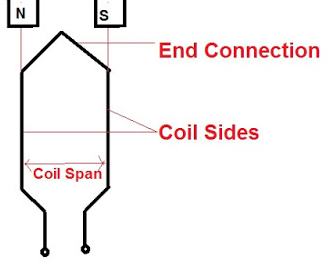# Coil Pitch or Coil Span, Full Pitch, Short Pitch Coil and Chording Angle

### Coil Pitch or Coil Span

Coil pitch or coil span is defined as the angular distance between the two coil sides of one coil. It is expressed in terms of electrical space degree. Coil pitch is 180 electrical space degree for full pitch coil and less than 180 degree for short pitch coil.

A coil is basically any number of turns. If there is only one turn, it is called single turn coil and if there is more than one turn then it is termed as multi-turn coil. A coil has two active length in which emf is generated. This active length of coil is called coil side. There are two coil sides in a single turn coil as can be seen from the figure below. Thus number of coil sides in a coil having N number of turn will be 2N. These coil sides of any phase are distributed in various slots of stator and connected in such a fashion that their induced emfs are additive in nature.

There are two types of coils: Full pitch coil and short pitch coil.

### Full Pitch Coil

When the angular distance between the two coil sides is one pole pitch, it is called full pitch coil. This means that both the coil sides are lying under the pole in full pitch coil. Figure below shows a full pitch coil.The electrical space angle between the active coil length or coil side is equal to one pole pitch. Since 1 pole pitch is the angular distance between the two consecutive poles which is equal to 180 electrical degree, therefore the coil span for full pitch coil is also equal to 180 electrical degree.

### Short Pitch Coil

If the angular distances between the two coil sides are less than one pole pitch, it is termed short pitch or fractional pitch coil. The coil span or coil pitch for short or fractional pitch coil is less than 180 electrical degree. In such coils, the two coil sides are not under the poles. Figure below shows a short pitched coil.In the above figure, it can be easily seen that the coil span is departing by some angle ε from 180 electrical space degree. This is called chording angle.

### Chording Angle

Chording angle can thus be defined as the angle by which coil span departs from the 180 electrical space degree. In the above figure, chording angle is ε and coil span is equal to (180-ε) space electrical degree. Chording angle is very important as pitch factor of coil is dependent on the chording angle. A proper selection of value of chording angle eliminates harmonics in the generated emf.

A short video to quickly understand the concept of coil pitch or coil span, Full Pitch & Short Pitch Coil and Chording Angle.

This site uses Akismet to reduce spam. Learn how your comment data is processed.